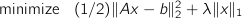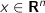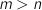# CVXGEN: Code Generation for Convex Optimization

## Example: Lasso

This example, from statistics, demonstrates the lasso procedure (-regularized least squares). For some more details, see Boyd and Vandenberghe,6.3.2.

## Optimization problem

We wish to solve the optimization problemwith parameters

•••and optimization variable

•The problem is interesting both when, and when.

## CVXGEN code

dimensions
m = 100
n = 10
end

parameters
A (m,n)
b (m)
lambda nonnegative  # regularization weight.
end

variables
x (n)
end

minimize